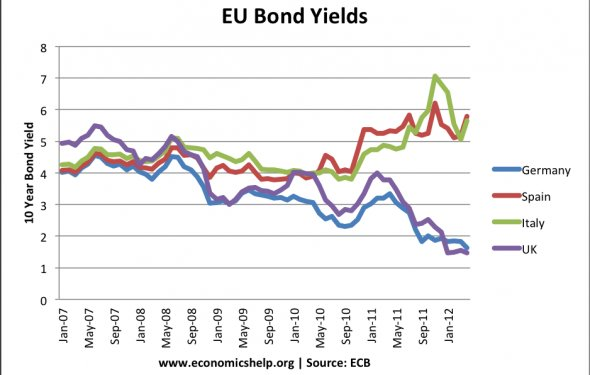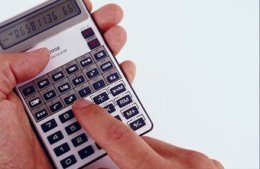# Market rate of bonds

How Do I Calculate the Market Price of a Bond?
June 28, 2017 – 01:31 pmCalculate the market value of a bond by comparing interest rates.

Calculator image by Alhazm Salemi from Fotolia.com

The market price of a bond is determined using the current interest rate compared to the interest rate stated on the bond. The market price of the bond comprises two parts. The first part is the present value of the bond's face value. The second part is the present value of the bond's interest payments. As an example, there is a \$100, 000 bond that pays interest semi-annually. The stated interest rate is 8 percent. The current market interest rate is 10 percent. The bond matures in five years.

1. Determine the interest payments by multiplying the interest rate per interest payment by the face value of the bond. In the example given, the interest rate per payment is 4 percent. This is half of the stated interest rate because the bond pays interest two times per year. Thus, 4 percent times \$100, 000 equals \$4, 000.

2. Determine the present value of an annuity factor for the interest payments. Use the present value of an annuity table. In the example, the term is 10, because the maturity is five years and the bond pays interest semi-annually. The interest rate is 5 percent, which is half of the current market rate, because the interest pays semi-annually. Using these figures, the present value of an annuity factor is 7.7217.

3. Multiply the interest payment by the present value of an annuity factor determined in Step 2. This is the present value of the interest payments. In the example, \$4, 000 times 7.7217 equals \$30, 886.80.

4. Determine the present value of \$1 factor. Use the present value of a \$1 table. In the example, the term is 10, because the maturity is five years and the bond pays interest semi-annually. The interest rate is 5 percent, which is half of the current market rate because the interest pays semi-annually. Using these figures, the present value of an annuity factor is 0.6139.

5. Multiply the face value of the bond by the present value of \$1 factor determined in Step 4. In the example, \$100, 000 times 0.6139 equals \$61, 390.

6. Add the present value of the interest payments, determined in Step 3, to the present value of the bond's face value, determined in Step 5. In the example, \$30, 886.80 plus \$61, 390 equals a bond market price of \$92, 276.80.